Latest Banking jobs   »   NIACL AO QUANT

# Quantitative Aptitude Quiz For NIACL AO Prelims 2023 -23rd August

Directions (1-5): In each of these questions, two equations are given. You have to solve both the equations and give answer
(a) if x>y
(b) if x≥y
(c) if x = y or no relation can be established between x and y.
(d) if y>x
(e) if y≥x

Q1. (i) x² – 12x + 32 = 0
(ii) y² – 20y + 96 = 0

Q2. (i) 2x² – 3x – 20 = 0
(ii) 2y² + 11y + 15 = 0

Q3. (i) x² – x – 6 = 0
(ii) y² – 6y + 8 = 0

Q4. (i) x² + 14x – 32 = 0
(ii) y² – y – 12 = 0

Q5. (i) x² – 9x + 20 = 0
(ii) 2y² – 12y + 18 = 0

Directions (6-10): In each of these questions, two equations (I) and (II) are given. You have to solve both the equations and give answer
(a) if x>y
(b) if x≥y
(c) if x<y
(d) if x ≤y
(e) if x = y or no relation can be established between x and y.

Q6. I. √625x² –375=0
II. √2116 y –736=0

Q7. I. 3x² + 5x + 2 = 0
II. 12y² + 11y + 2 = 0

Q8. I. 16x + 11y = 154
II. 11x + 16y = 89

Q9. I. 3x² + 14x – 5 = 0
II. 3y² – 19y + 6 = 0

Q10. I. 7x² – 11x – 6 = 0
II. 7y² – 10y + 3 = 0

Solutions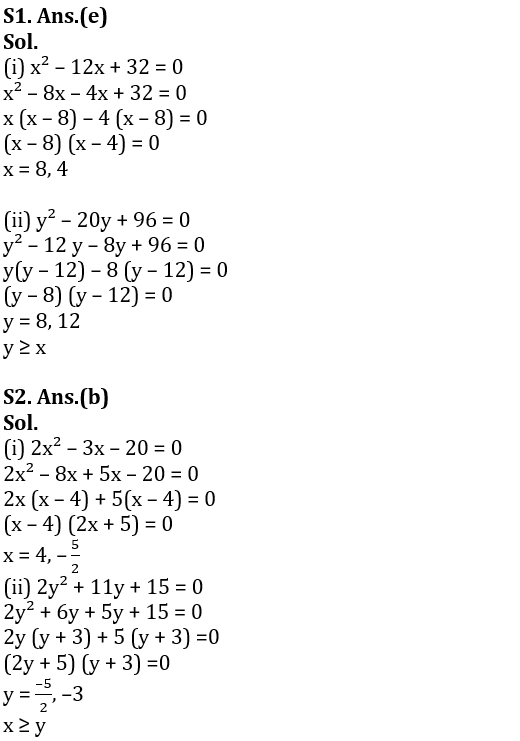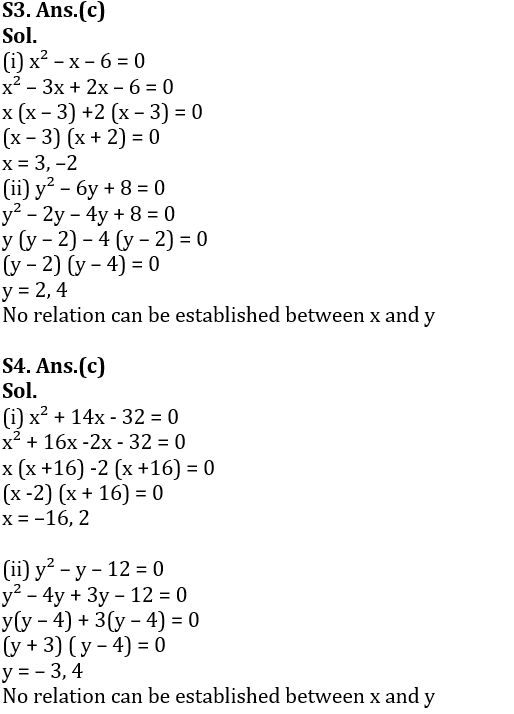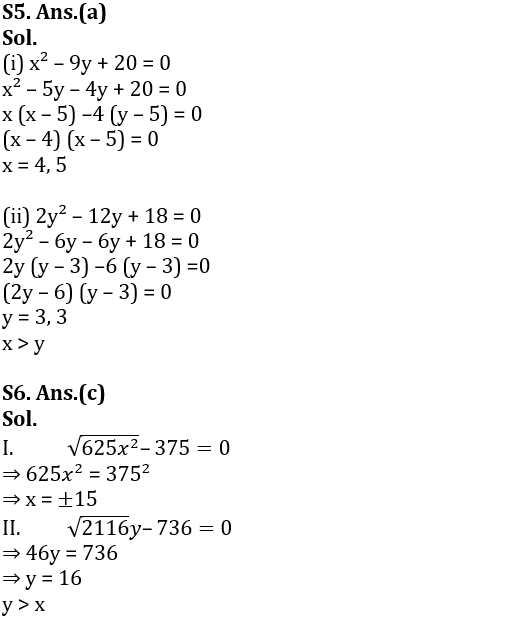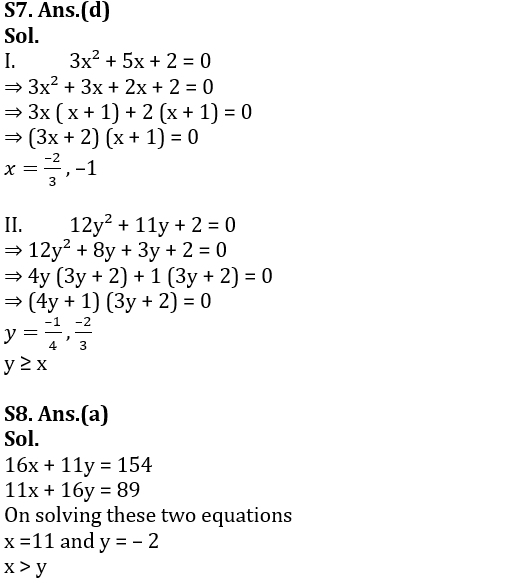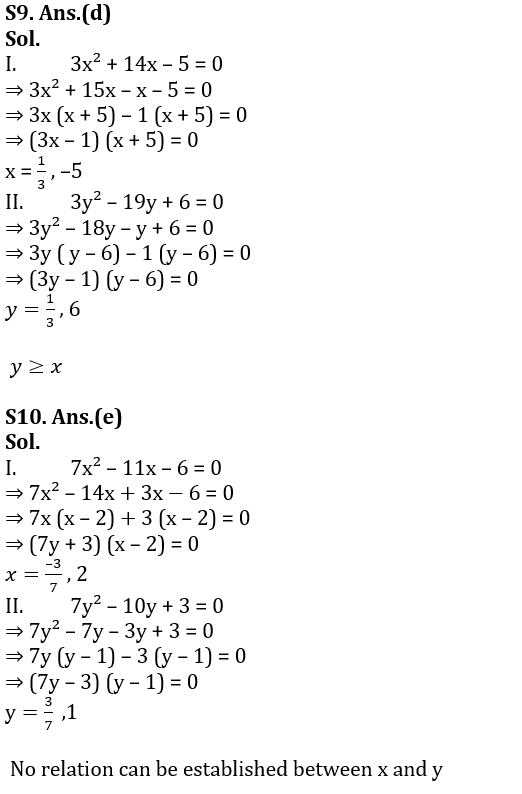.                                    .                                                .                .

## FAQs

### What are the subjects asked in NIACL AO Prelims exam 2023?

Subjects asked in NIACL AO Prelims are Reasoning Ability

#### Congratulations!Union Budget 2023-24: Free PDF